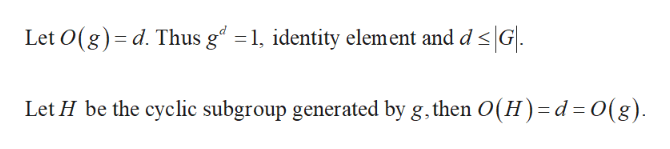# 9*. Use Lagrange's theorem to prove: If g E G, G a finite group, then gG| = 1.

Question
1 viewshelp_outlineImage Transcriptionclose9*. Use Lagrange's theorem to prove: If g E G, G a finite group, then gG| = 1. fullscreen
check_circle

Step 1

To prove the given statement,...help_outlineImage Transcriptioncloseidentity element and d s G Let O(g) d. Thus g2 1 Let H be the cyclic subgroup generated by g, then O(H)=d = O(g) fullscreen

### Want to see the full answer?

See Solution

#### Want to see this answer and more?

Solutions are written by subject experts who are available 24/7. Questions are typically answered within 1 hour.*

See Solution
*Response times may vary by subject and question.
Tagged in

### Math